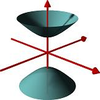#### You may also like### A Close Match

Can you massage the parameters of these curves to make them match as closely as possible?### Prime Counter

A short challenge concerning prime numbers.### The Right Volume

Can you rotate a curve to make a volume of 1?

# Quick Sum

##### Age 16 to 18 ShortChallenge Level

According to my calculator, I see that

$$\frac{1}{\sqrt{1}+ \sqrt{2}}+ \frac{1}{\sqrt{2}+ \sqrt{3}} + \text{and so on up to}+\frac{1}{ \sqrt {15}+ \sqrt{16}} = 3.0000000000$$
How could I prove that the answer is, indeed, exactly 3?

Did you know ... ?

Mathematicians often use calculating aids to help form an opinion concerning the likely numerical answer to a problem involving irrational numbers, but will always seek a full proof which does not rely on the calculator to complete the problem.